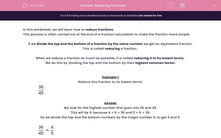# Reducing Fractions

In this worksheet, students use common factors to simplify fractions.Key stage:  KS 2

Curriculum topic:   Maths and Numerical Reasoning

Curriculum subtopic:   Fractions

Difficulty level:#### Worksheet Overview

In this worksheet, we will learn how to reduce fractions

This process is often carried out at the end of a fraction calculation to make the fraction more simple.

If we divide the top and the bottom of a fraction by the same number we get an equivalent fraction.

This is called reducing a fraction.

When we reduce a fraction as much as possible, it is called reducing it to its lowest terms.

We do this by dividing the top and the bottom by their highest common factor.

Example 1

Reduce this fraction to its lowest terms.

 36 45

We look for the highest number that goes into 36 and 45.

This will be 9, because 4 × 9 = 36 and 5 × 9 = 36.

So we divide the top and the bottom numbers by the magic number 9, to get 4 and 5.

 36 = 4 45 5

We cannot reduce this any further, so the answer is:

 4 5

Example 2

Reduce this fraction to its lowest terms.

 64 96

Sometimes it is hard to find the highest common factor.

We look for any number that goes into 64 and 96.

This could be 8, because 8 × 8 = 64 and 12 × 8 = 96.

So we divide the top and the bottom numbers by the magic number 8, to get 8 and 12.

 64 = 8 96 12

Now we reduce again by looking for the highest number that goes into 8 and 12.

This is 4, because 2 × 4 = 8 and 3 × 4 = 12.

So we divide the top and the bottom numbers by the magic number 4, to get 2 and 3.